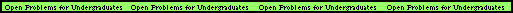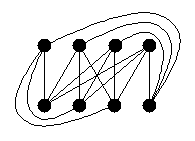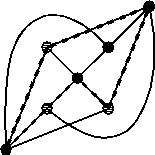# Graph Theory Open Problems

Index of Problems

### Unit Distance Graphs---chromatic number

RESEARCHER: Robert Hochberg
OFFICE: CoRE 414
Email:hochberg@dimacs.rutgers.edu
DESCRIPTION: How many colors are needed so that if each point in the plane is assigned one of the colors, no two points which are exactly distance 1 apart will be assigned the same color? This problem has been open since 1956. It is known that the answer is either 4, 5, 6 or 7---this is not too hard to show. You should try it now in order to get a flavor for what this problem is really asking. This number is also called ``the chromatic number of the plane.''

A graph which can be embedded in the plane so that vertices correspond to points in the plane and edges correspond to unit-length line segments is called a ``unit-distance graph.'' The question above is equivalent to asking what the chromatic number of unit-distance graphs can be.

Here are some warm-up questions, whose answers are known: What complete bipartite graphs are unit-distance graphs? What's the smallest 4-chromatic unit-distance graph? Show that the Petersen graph is a unit-distance graph.### Unit Distance Graphs---girthRESEARCHER: Robert Hochberg
OFFICE: CoRE 414
Email:hochberg@dimacs.rutgers.edu
DESCRIPTION: As the problem mentioned above remains unsolved, mathematicians have turned their attention to related problems in the hopes of gaining some insight into this difficult question.

In this problem, we are interested in finding what sort of unit-distance graphs we can make--in particular, can we find 4-chromatic graphs which have large girth? Paul O'Donnell has found a unit distance graph of girth 12 which cannot be 3-colored, but this graph has an incredibly large number of points. Hochberg and O'Donnell have found 4-chromatic unit-distance graphs of girths 4 and 5 with 23 (shown to the right) and 45 vertices respectively. Are there smaller ones? Are there 4-chromatic unit-distance graphs of arbitrarily large girth? An answer to this question may help to solve the "chromatic number of the plane" problem.### Barnette's ConjectureRESEARCHER: Peter Hamburger
OFFICE: CoRE 442
Email:hamburge@dimacs.rutgers.edu
DESCRIPTION: Is it true that every 3-connected, 3-regular, planar bipartite graph is Hamiltonian?

It is known that this is not true if you remove the "bipartite" condition, but the smallest known such graph which is not Hamiltonian has 38 vertices, as shown to the right.### Crossing Number of K(9, 9)RESEARCHER: Robert Hochberg
OFFICE: CoRE 414
Email:hochberg@dimacs.rutgers.edu
DESCRIPTION: What is the crossing number of the complete bipartite graph K(9, 9)? It is conjectured to be 256, but nobody knows.

Another way to ask this is the following: If you place 9 red points in the plane and 9 blue points in the plane, and then connect each red point to each blue point with curves (81 curves in all), then what is the minimum number of crossing points that must appear in your drawing?

An example for K(4,4) is shown to the right, with 8 crossings. Actually, this graph can be drawn with just 4 crossings...can you find it?

For these drawings, it is assumed that the curves do not touch the points (vertices) except at their endpoints, and that no three curves meet at a point.### Vertices and Neighbors on a CycleRESEARCHER: Nate Dean
OFFICE: Chair of Mathematics, Texas Southern University
Email: dean_nx@tsu.edu
DESCRIPTION: Is it true that every k-connected (k>1) graph which does not have a Hamiltonian cycle has a cycle that contains k independent vertices and their neighbors? This is known to be true for k = 2 and 3. For example, the graph to the right is 3-connected but not Hamiltonian. And the dotted cycle shown contains 3 independent vertices (the three vertices which are lighter in color) and thier neighbors. To see that it is not Hamiltonian, notice that this graph is just the complete bipartite graph K(3,4).### The Square of a Directed Graph

RESEARCHER: Nate DeanOFFICE: Chair of Mathematics, Texas Southern University
Email: dean_nx@tsu.edu
DESCRIPTION: This problem deals with the "square of an oriented graph." An oriented graph is a simple graph (no loops or multiple edges) in which each edge is replaced by an arc. Thus you produce a simple directed graph without pairs of "reversed arcs."

To get the square of an oriented graph (or any directed graph) you leave the vertex set the same, keep all the arcs, and for each pair of arcs of the form (u,v), (v,w), you add the arc (u,w) if that arc was not already present. An example of an oriented graph and its square is shown above.

Here is the open problem: Prove that for every oriented graph, D, there exists a vertex whose out-degree at least doubles when you square the oriented graph. In the example above, the vertices A, B, C, E and G satisfy this property. (For vertices A and G, 2*0=0). Nate learned of this problem from Paul Seymour. David Fisher proved this theorem for tournaments, i.e., orientations of complete graphs.

